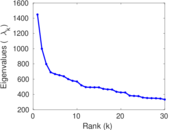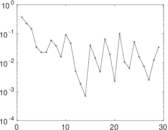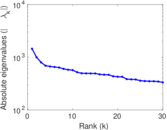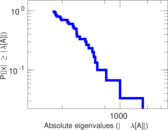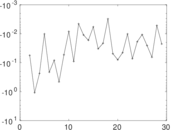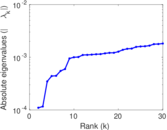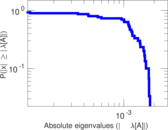# CiteULike user–item

This is the bipartite network of users and publications in CiteULike. Each edge represents a tag assignment that connects a users and a publication. Since a user can be assign multiple tags to a publication, the network contains multiple edges. The edges are annotated with the creation time of the tag assignment.

 Code `Cui` Internal name `citeulike-ui` Name CiteULike user–item Data source http://www.citeulike.org/faq/data.adp AvailabilityDataset is available for download Consistency checkDataset passed all tests Category Interaction network Dataset timestamp 2007 Node meaning User, publication Edge meaning Tag assignment Network formatBipartite, undirected Edge typeUnweighted, multiple edges Temporal dataEdges are annotated with timestamps

## Statistics

 Size n = 754,484 Left size n1 = 22,715 Right size n2 = 731,769 Volume m = 2,411,819 Unique edge count m̿ = 842,426 Wedge count s = 343,677,541 Claw count z = 476,035,640,534 Cross count x = 909,343,585,975,693 Square count q = 962,735 4-Tour count T4 = 1,384,098,060 Maximum degree dmax = 57,706 Maximum left degree d1max = 57,706 Maximum right degree d2max = 1,646 Average degree d = 6.393 29 Average left degree d1 = 106.177 Average right degree d2 = 3.295 87 Fill p = 5.068 10 × 10−5 Average edge multiplicity m̃ = 2.862 94 Size of LCC N = 576,245 Diameter δ = 20 50-Percentile effective diameter δ0.5 = 6.265 75 90-Percentile effective diameter δ0.9 = 8.501 64 Median distance δM = 7 Mean distance δm = 7.064 48 Gini coefficient G = 0.747 683 Balanced inequality ratio P = 0.209 926 Left balanced inequality ratio P1 = 0.128 030 Right balanced inequality ratio P2 = 0.303 091 Relative edge distribution entropy Her = 0.848 102 Power law exponent γ = 9.072 94 Tail power law exponent γt = 3.281 00 Tail power law exponent with p γ3 = 3.281 00 p-value p = 0.000 00 Left tail power law exponent with p γ3,1 = 1.461 00 Left p-value p1 = 0.000 00 Right tail power law exponent with p γ3,2 = 3.321 00 Right p-value p2 = 0.061 000 0 Degree assortativity ρ = −0.023 376 8 Degree assortativity p-value pρ = 3.765 43 × 10−102 Spectral norm α = 1,448.25 Algebraic connectivity a = 0.000 109 746 Spectral separation |λ1[A] / λ2[A]| = 1.448 50 Controllability C = 709,624 Relative controllability Cr = 0.940 542

## Plots

### Degree distribution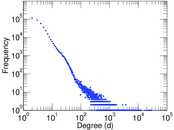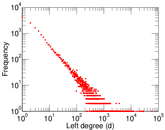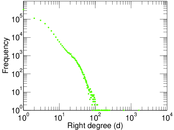### Cumulative degree distribution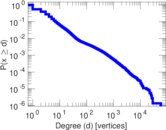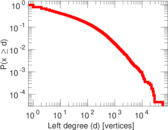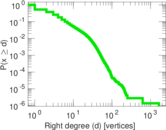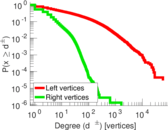### Lorenz curve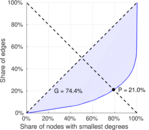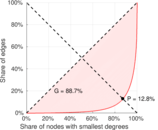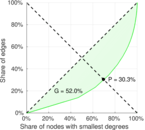### Spectral distribution of the adjacency matrix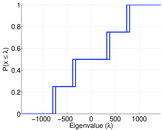### Spectral distribution of the normalized adjacency matrix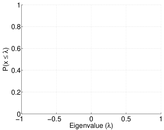### Spectral distribution of the Laplacian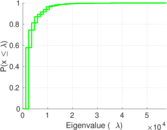### Spectral graph drawing based on the adjacency matrix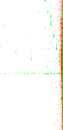### Spectral graph drawing based on the Laplacian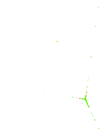### Spectral graph drawing based on the normalized adjacency matrix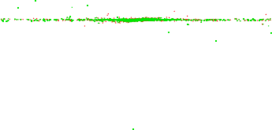### Degree assortativity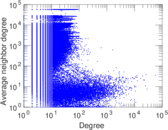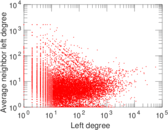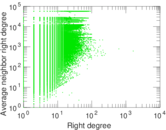### Zipf plot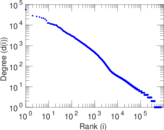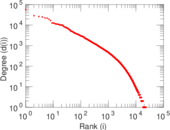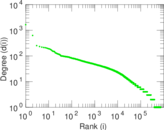### Hop distribution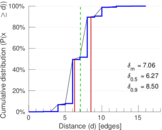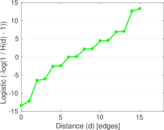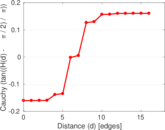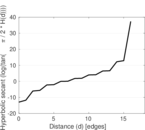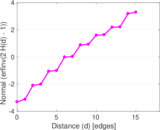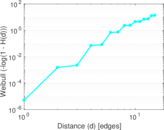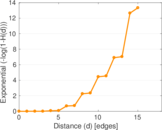### Delaunay graph drawing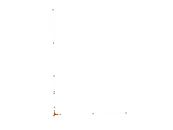### Edge weight/multiplicity distribution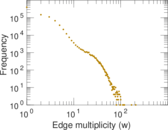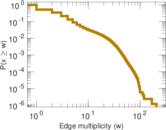### Temporal distribution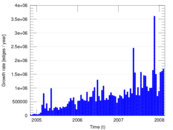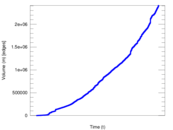### Diameter/density evolution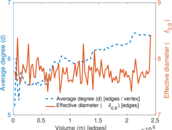### Inter-event distribution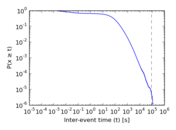### Node-level inter-event distribution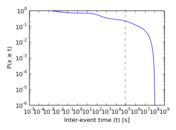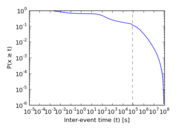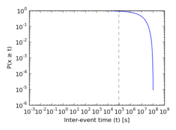### Matrix decompositions plots